## Main

Imagine air molecules in a room. They move around with all possible velocities in all directions. Attaining the exact knowledge of these trajectories is a daunting and an unrealistic task. Statistical mechanics, however, claims that exact knowledge of individual trajectories is not required and systems can be accurately described using only a few parameters. What is the essential property of these systems that allows for such a simple description?

Ergodic dynamics provide an explanation for this simplicity. If the dynamics are ergodic, then the system will uniformly explore all microscopic states over time, constrained only by conservation laws. Ergodicity ensures that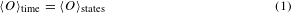where O is any macroscopic observable and brackets denote averaging. In the limit of many particles, macroscopic observables such as pressure or density approach equilibrium and are stationary—therefore, at any time, O(t) = 〈Otime. These two equations imply that macroscopic observables can be predicted by uniformly averaging over all states, and this forms the foundation for all thermodynamic calculations.

In classical systems, it is chaotic motion which drives the system to ergodically explore the state space5. Quantum systems, however, are governed by Schrodinger’s equation, which is linear and consequently forbids chaotic motion6. This poses fundamental questions regarding the applicability of statistical mechanics in isolated quantum systems1,2,3,4. Do quantum systems exhibit ergodic behaviour in the sense of equation (1)? Do quantum systems act as their own bath to approach thermal equilibrium? Extensive experimental efforts have been made to address these fundamental questions7,8,9,10,11,12,13.

Here, we investigate ergodic dynamics by considering a simple quantum model whose classical limit is chaotic14,15,16,17,18. This model describes a collection of spin-1/2 particles whose collective motion is equivalent to that of a single larger spin with total angular momentum j governed by the Hamiltonian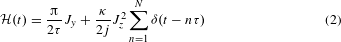where Jy and Jz are angular momentum operators. The sum over delta functions implies N applications of Jz2, each at integer time steps. The angular momentum operators can be expressed in terms of the constituent spin-1/2 Pauli operators, for example, Jz = (/2)∑ iσz(i). Setting τ = 1, the first term in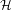causes each spin to rotate around the y-axis by an angle π/2. The second term couples every spin to every other spin with strength κ/2j. This can be seen by expanding Jz2 in terms of z operators, where terms such as σz(1)σz(2) and all other combinations appear.

The classical dynamics, being simple to visualize and interpret, can provide valuable intuition for studying the quantum limit. The classical limit of this model occurs when j is very large and quantization effects become negligible. In this limit, the system behaves like a classical spinning top, with dynamics which are known to be chaotic14,15,16,17. The parameter κ sets the chaoticity and takes the dynamics from regular to chaotic as κ increases; at intermediate values, the system exhibits a rich mixture of both regular and chaotic motion.

Experimentally realizing this model requires a high degree of control over both local terms and interactions in a multi-qubit Hamiltonian. This led to the design of a three-qubit ring of planar transmons with tunable inter-qubit coupling (see Supplementary Information)19,20,21. The rotations around the y-axis (Jy) are performed using shaped microwave pulses that are resonant with the qubit transition. The simultaneous and symmetric three-qubit interaction (Jz2) is turned on and off using a tunable coupling circuit controlled by three separate square pulses. The qubit–qubit interaction energy g and the duration of the interaction pulses T set κ through the relation κ = 3gT/. We measure the strength of the interaction energy κ by determining the time it takes for an excitation to swap between the qubits (see Supplementary Information).

The periodic nature ofallows us to write down the unitary evolution over one cycle as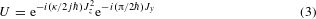shown schematically in Fig. 1a. We begin by initializing each qubit in the state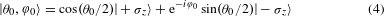where θ0 and φ0 are angles describing the orientation of the single-qubit states. This state is known as a spin coherent state and is the most classical spin state in the sense of minimum uncertainty and zero entanglement. After preparing the initial state, we rotate each qubit around the y-axis by an angle π/2. Next, we allow all of the qubits to interact with one another for a duration which sets the value of κ. We repeat these two steps N times and then tomographically reconstruct the resulting density matrix22. For details regarding the pulse sequence, see Supplementary Information.

We visualize the evolution of the system by depicting the single-qubit state as a vector inside of a Bloch sphere, shown in Fig. 1b. Each Bloch vector is constructed by measuring the expectation values of the x, y and z Pauli operators after evolving the system according to equation (3). As the dynamics are symmetric under qubit exchange, the qubits undergo nominally identical evolution and we plot the average behaviour (see Supplementary Information). The chosen initial state is shown in red, with the Bloch vector after subsequent steps shown in blue. After each step, there are two qualitative changes: a rotation and a change in the length. The orientation is analogous to the orientation of the classical spin. The change in length, however, describes entanglement among the qubits.

Entanglement can be characterized using the entanglement entropy S,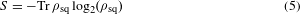where ρsq is the density matrix of a single qubit. Writing the trace as a sum reproduces the familiar definition of entropy −∑ pi log(pi), where pi is the probability of being in the ith microstate. If the qubit is in a pure state, then the single-qubit state is completely known and the entropy is zero. However, if the qubits are entangled with one another, then ρsq is a statistical mixture of states and the entropy is non-zero.

In Fig. 2a, we show the entanglement entropy between a single qubit and the rest of the qubits at several instances in time. The entanglement entropy is measured by performing state tomography on the individual qubits22 and then directly applying equation (5) to the reconstructed density matrix. In each panel, we prepare various initial states |θ0, φ0〉, evolve the system for N steps and plot the entanglement entropy; different panels correspond to different N. Initial states prepared close to the y-axis have low entropy (red), which remains low as the system evolves. States prepared farther away from the y-axis gain higher entropy (blue) given sufficient time. We perform the same set of experiments for stronger interaction, κ = 2.5, shown in Fig. 2b. At stronger interactions, the entropy increases rapidly and regions of low entropy are no longer isolated to near the y-axis.

In Fig. 2a, b, we see that the entropy fluctuates over time. In small quantum systems, there are fluctuations or revivals that vanish when the system size is taken to infinity (known as the thermodynamic limit). For finite systems, averaging the entropy over time is commonly used to estimate the equilibrium value approached by larger systems. In Fig. 2c, we show the entanglement entropy averaged over time (N) for both values of interaction strength κ. The corresponding classical dynamics are shown in Fig. 2d.

We find a striking resemblance between the average entanglement in the quantum system and chaotic dynamics in the classical limit. The regions of classical phase space where the dynamics are chaotic correspond to high entropy (blue) in the quantum system; regions that are classically regular correspond to low entropy (red), including bifurcation at large κ. The results shown in Fig. 2b have been predicted by recent theoretical works23,24. The connection between chaos and entanglement has been of theoretical interest for a considerable time25,26,27. However, these studies focused on very large systems near the border of quantum and classical physics28,29. Here, we show that the results hold deep in the quantum limit. It is interesting to note that chaos and entanglement are each exclusive to their respective classical and quantum domains, and any connection is surprising. The correspondence is even more unexpected given that our system is so far from the classical limit6,30.

In Fig. 2b, the entanglement entropy in the blue regions approaches 0.8, close to the maximum attainable value of 1.0 for a single qubit. The value of 0.8 is very close to the value one would obtain by uniformly averaging over all states, 0.73 (ref. 31). In equation (2), the Hamiltonian depends on time and, as a result, energy is not conserved. Therefore, statistical mechanics would predict the values of observables using an ensemble with maximum entropy or, equivalently, an infinite temperature ensemble. The observed density matrix approaching maximum entropy suggests that even small quantum systems undergoing unitary dynamics can appear to thermalize3,32,33.

In the Supplementary Information, we numerically compute the evolution for larger systems and show that fluctuations decrease with increasing system size, as expected for finite-size systems approaching thermal equilibrium. Additionally, we compute the behaviour at larger values of κ and show that all initial states obtain near-maximal entropy, as opposed to the mixed phase space shown in Fig. 2. This further supports the idea that what we see in the experiment is the onset of thermalization in a small quantum system.

The observed single-qubit entropy can originate from two sources: entanglement with the other qubits and entanglement with the environment (decoherence). In Fig. 3, we show that the contrast between high- and low-entropy results from entanglement among the qubits, confirming our assumption that the system is well isolated. To distinguish these two effects, we measure the three-qubit density matrix. Using these measurements, we compute the expectation values of all combinations of Pauli operators. The first nine columns in Fig. 3 contain operators on only a single qubit, and thus provide information about local properties. The remaining columns contain products of two- and three-qubit operators, and describe correlations between the qubits.

In the top panel, we consider an initial state whose entropy has increased by the least amount (most red), shown inset. After ten time steps, we see that each qubit is oriented along the y-axis, as indicated by the first three peaks. The qubits pointing along the same direction lead to classical correlations, as indicated by the remaining peaks among the two- and three-qubit correlations. In the lower panel, we consider an initial state whose entropy has increased by the largest amount (most blue). In addition to the qubit orientations and classical correlations, we also find many significant peaks among the multi-qubit correlations. These non-classical correlations are clear signatures of entanglement among the qubits. Additionally, we find that the three-qubit state purity, a measure of decoherence, is equal for both of these states, showing that the contrast between high and low entropy is entirely the result of inter-qubit entanglement (see Supplementary Information). This finding is in contrast to previous studies which found that, for initial states with high entropy, the system displayed a hypersensitivity to perturbations, such as environmental decoherence17.

The advantage of studying statistical mechanics in a small quantum system is that we can directly check for ergodic motion in the three-qubit dynamics. Using measurements of the full multi-qubit density matrix, we investigate the connection between ergodic dynamics in the full system and entropy production in subsystems. Note that the full system is ideally in a pure state whose entropy is zero and stays zero as the system evolves—this is in stark contrast to subsystems which gain entropy over time through entanglement. While the full system cannot thermalize in the sense of reaching maximum entropy, it can undergo ergodic motion (time averages being equal to state-space averages). In statistical mechanics, a uniform average over states is given by the microcanonical ensemble. In Fig. 4, we plot the overlap of the time-averaged density matrix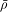with a microcanonical ensemble ρmc, given by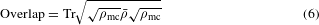Here, ρmc is an 8-by-8 density matrix which attributes equal probability to all of the accessible states. The overlap of these two distributions approaching 1.0 would imply that time averages are equivalent to state-space averages for all measurable quantities.